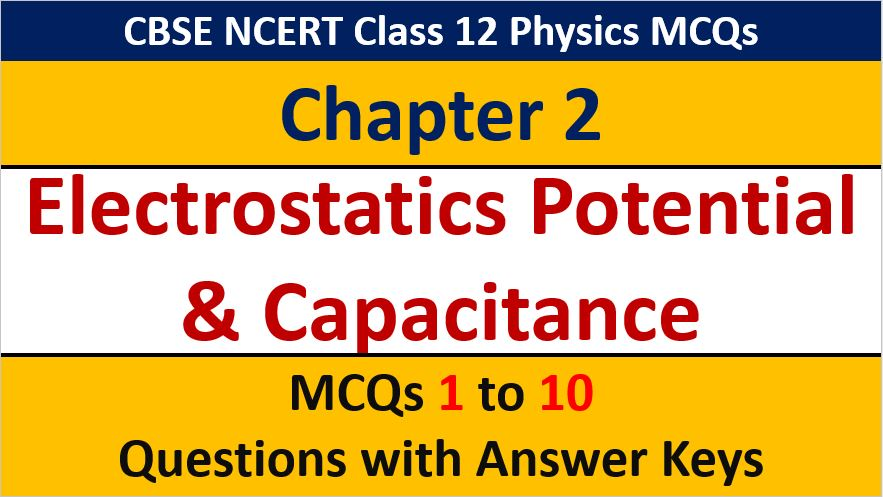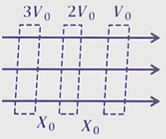## MCQ Questions for Class 12 Physics Chapter 2 Electrostatics Potential and Capacitance with Answer Keys

If you are preparing for CBSE Class 12 Board Exam Term 1 which will be an objective type questions paper, then you need to practice multiple choice questions of Class 12 Physics Chapterwise. In this article of AKVTutorials, you will get MCQ Questions for Class 12 Physics Chapter 2 Electrostatics Potential and Capacitance with Answer Keys.

MCQ Questions for Class 12 Physics Chapter 2 Question No 1

A unit charge is taken from one point to another over an equipotential surface, then

Option A : Work is done on the charge

Option B : Work is done by the charge

Option C : Work on the charge is constant

Option D : No work is done

Option D : No work is done

MCQ Questions for Class 12 Physics Chapter 2 Question No 2:

Equipotential surface are show in figure a and b, the field in

Figure a:Figure b:Option A : a is uniform only

Option B : b is uniform only

Option C : a and b is uniform

Option D : both are non uniform

Option A : a is uniform only

MCQ Questions for Class 12 Physics Chapter 2 Question No 3

When the separation between two charges is increased the electric potential energy of the charges

Option A : Increases

Option B : Decreases

Option C : Remains the same

Option D : May increase or decrease

Option D : May increase or decrease

MCQ Questions for Class 12 Physics Chapter 2 Question No 4:

Potential at the point of a pointed conductor is

Option A : Maximum

Option B : Same as at any other point

Option C : Zero

Option D : Minimum

Option B : Same as at any other point

MCQ Questions for Class 12 Physics Chapter 2 Question No 5:

If two conducting spheres are separately charged and then brought into contact

Option A : The total energy of the sphere is conserved

Option B : The total charge on the spheres is conserved

Option C : Both the total energy and charge are conserved

Option D : The final potential is always the mean of the original potential of the two spheres

Option B : The total charge on the spheres is conserved

MCQ Questions for Class 12 Physics Chapter 2 Question No 6:

On the perpendicular bisector of an electric dipole, the electric intensity E and potential V are,

Option A : E = 0, V = 0

Option B : E ≠ 0, V ≠ 0

Option C : E ≠0, V = 0

Option D : E ≠ 0, V ≠ 0

Option C : E ≠0, V = 0

MCQ Questions for Class 12 Physics Chapter 2 Question No 7:

When an electron approaches a proton, their electrostatic potential energy

Option A : Decreases

Option B : Increases

Option C : Remains unchanged

Option D : All the above

Option D : Decreases

MCQ Questions for Class 12 Physics Chapter 2 Question No 8:

If an earthed place is brought near positively charged plate, the potential and capacity plate

Option A : Increases, Decreases

Option B : Decreases, Increases

Option C : Decreases, Decreases

Option D : Increases, Increases

Option B : Decreases, Increases

MCQ Questions for Class 12 Physics Chapter 2 Question No 9:

An electron of mass m and charge e is accelerated from rest through a potential difference V in vacuum. Its final speed will be

Option A : √2ev / m

Option B : √ev / m

Option C : ev / 2m

Option D : ev / m

Option A : √2ev / m

MCQ Questions for Class 12 Physics Chapter 2 Question No 10:

Identify the correct order in which the gain in kinetic energies increases in the following cases

Ⅰ. Alpha particle accelerated through  P.D of 2V

Ⅱ. Proton accelerated through a P.D of 2V

Ⅲ. Deutron accelerated through a P.D of 3V

Ⅳ. Electron accelerated through a P.D of 5V

Option A ,  Ⅲ , Ⅰ and  Ⅳ

Option B Ⅲ, Ⅳ, Ⅰ and Ⅱ

Option C :  Ⅳ, Ⅱ, Ⅰ and  Ⅲ

Option D : Ⅰ, Ⅲ , Ⅱ and Ⅳ

Option A : Ⅱ,  Ⅲ , Ⅰ and  Ⅳ

CBSE Class 12 Physics Chapterwise MCQ
1 : Electric Charge & Fields MCQs
| 1 to 10 | 11 to 20
2 : Electrostatics Potential and Capacitance MCQs
| 1 to 10 | 11 to 20
3 : Current Electricity MCQs
| 1 to 10 | 11 to 20
4 : Moving charges and Magnetism MCQs
| 1 to 10 | 11 to 20
5 : Magnetism and Matter MCQs
| 1 to 10 | 11 to 20
6 : Electromagnetic Induction MCQs
| 1 to 10 | 11 to 20
7 : Alternating Current MCQs
| 1 to 10 | 11 to 20
8 : Electromagnetic Waves MCQs
| 1 to 10 | 11 to 20
9 : Ray Optics and Optical Instruments MCQs
| 1 to 10 | 11 to 20
10 : Wave Optics MCQs
| 1 to 10 | 11 to 20
11 : Dual Nature of Radiation MCQs
| 1 to 10 | 11 to 20
12 : Atoms MCQs
| 1 to 10 | 11 to 20
13 : Nuclei MCQs
| 1 to 10 | 11 to 20
14 : Semi Conductor Electronics MCQs
| 1 to 10 | 11 to 20
15 : Communication Systems MCQs
| 1 to 10 | 11 to 20